# Ask Ethan: Why Do Gravitational Waves Travel E…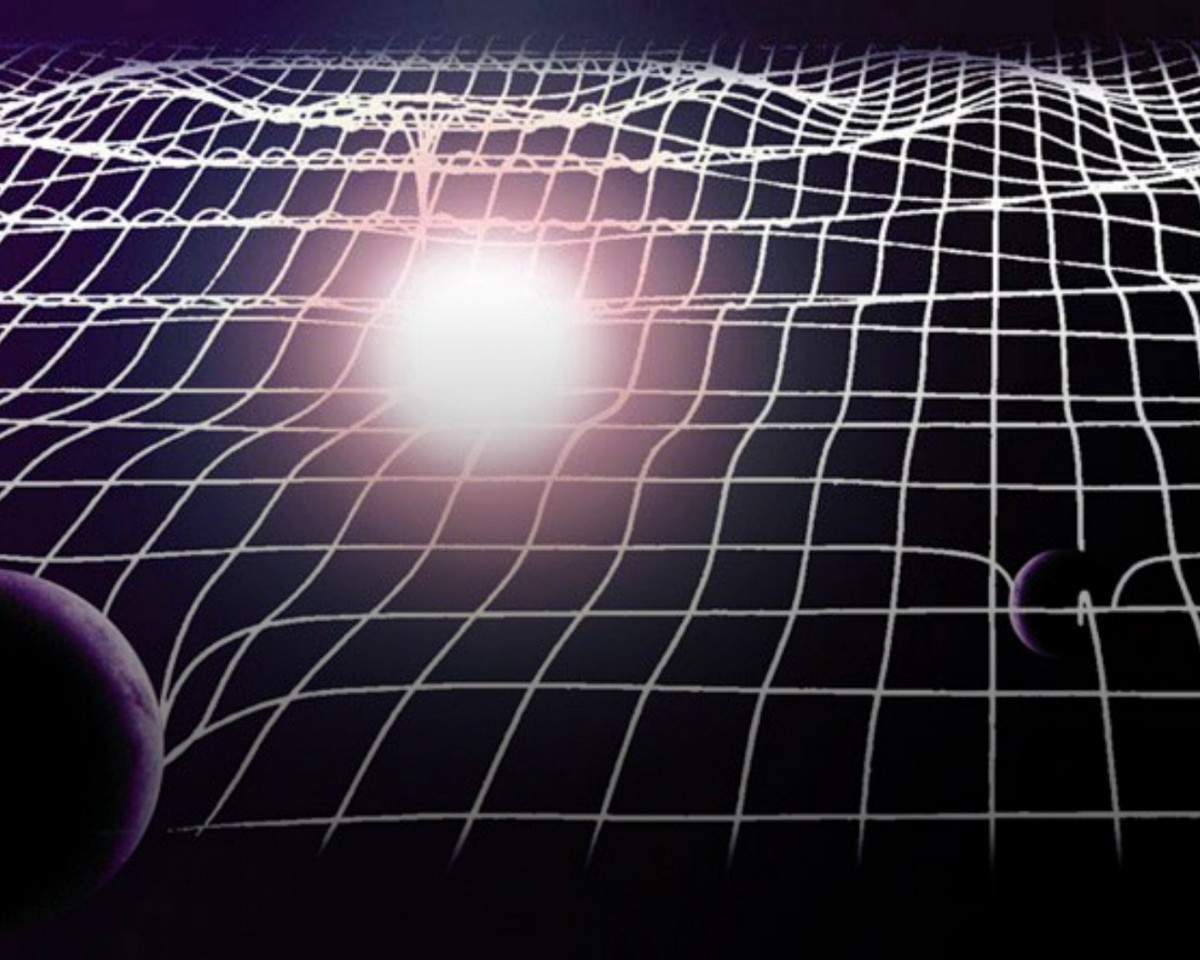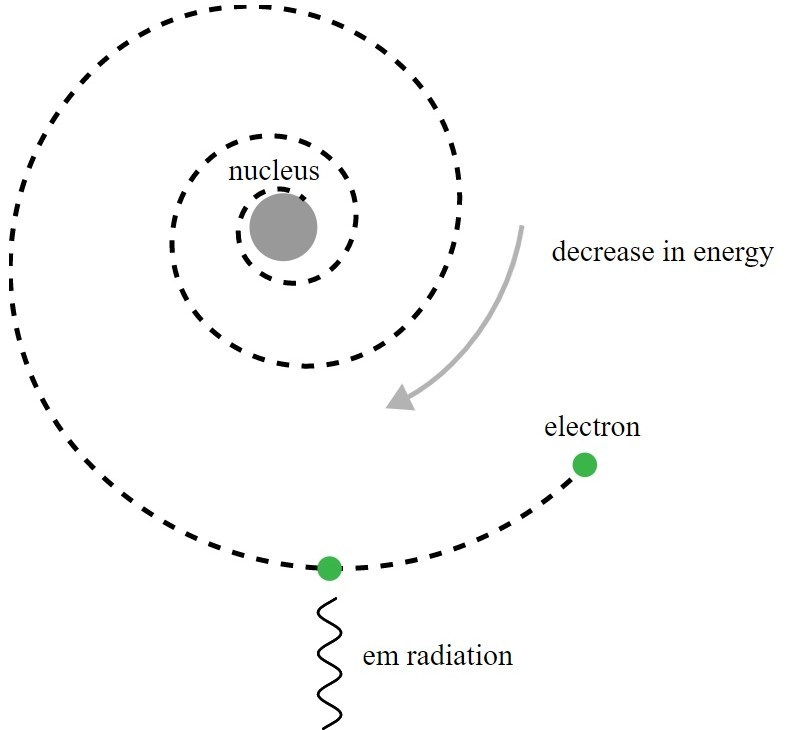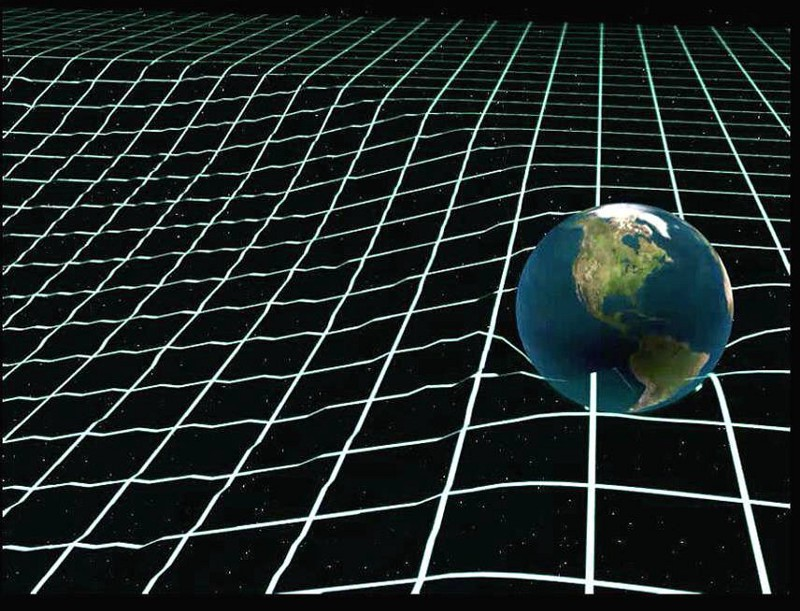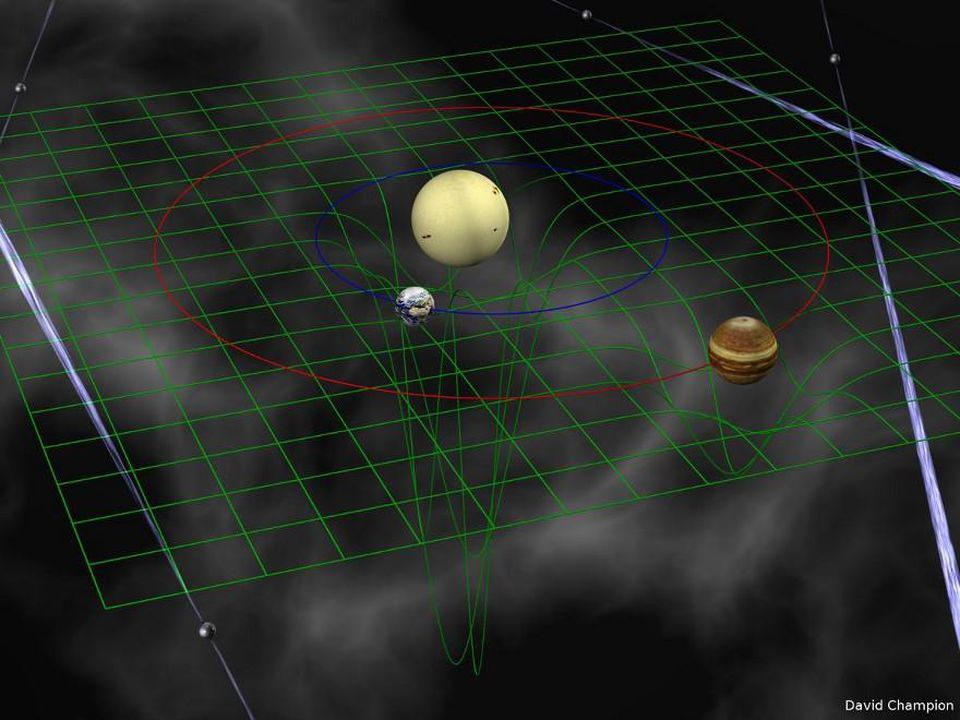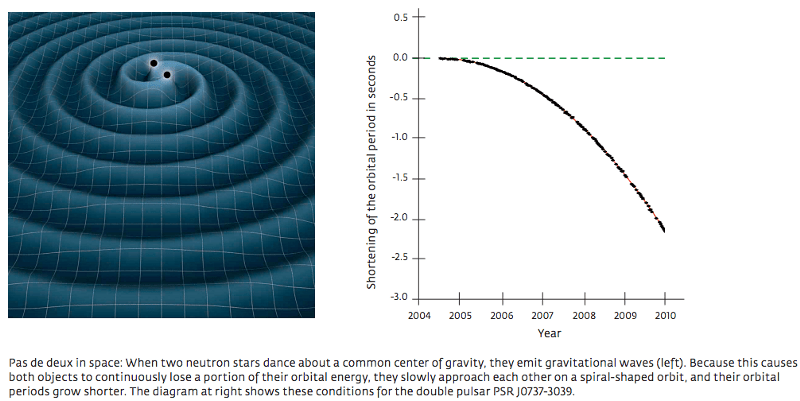## Ask Ethan: Why Do Gravitational Waves Travel Exactly At The Speed Of Light?

We know that the speed of electromagnetic radiation can be derived from Maxwell’s equation[s] in a vacuum. What equations (similar to Maxwell’s – perhaps?) offer a mathematical proof that Gravity Waves must travel [at the] speed of light?

If you were to somehow make the Sun disappear, you would still see its emitted light for 8 minutes and 20 seconds: the amount of time it takes light to travel from the Sun to the Earth across 150,000,000 km of space. But what about gravitation? Would the Earth continue to orbit where the Sun was for that same 8 minutes and 20 seconds, or would it fly off in a straight line immediately?

There are two ways to look at this puzzle: theoretically and experimentally/observationally. From a theoretical point of view, this represents one of the most profound differences from Newton’s gravitation to Einstein’s, and demonstrates what a revolutionary leap General Relativity was. Observationally, we only had indirect measurements until 2017, where we determined the speed of gravity and the speed of light were equal to 15 significant digits!

Gravitational waves do travel at the speed of light, which equals the speed of gravity to a better precision than ever. Here’s how we know.